# 1,20 % p.a. is the maximal interest rate under the business term deposits for 3 months12.08.2020

## Bankchart.com.au has surveyed the terms of obtaining business term deposits for 3 months in the national currency. The survey covered all the deposits with any form of interests repayment, regardless of the replenishment option or the deposit partial withdrawal option. Our website's analysts have studied the deposit offers from all Australian banks. Review date - 12.08.2020

As of 28 July 2020 the deposits under review have been offered by 19 banks.  The average interest rate under such deposits equals 0,53 % p.a. The total number of the deposits programs available for the specified terms is 45.

The maximal return among the deposits under review is offered by RACQ. The interest rate under "Term Deposit" deposit program constitutes 1,20 % p.a. The table below shows the best offers by each bank selected by Bankchart.com.au (in terms of profitability) for the aforementioned terms.

### The TOP-19 business term deposits for 3 months, as of 28 July 2020

#

Name

Interest Rate

1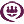RACQ

«Term Deposit»

1,20

interest rate, %

rate type

fixed

interest periodicity

monthly

minimum sum

\$ 1 000,00

2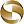Mega International Commercial Bank

1,00

interest rate, %

rate type

fixed

interest periodicity

at maturity

minimum sum

\$ 1 000,00

3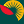Suncorp Bank

0,90

interest rate, %

rate type

variable

interest periodicity

monthly

minimum sum

\$ 0,00

4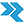Rural Bank

«Fixed rate FMD»

0,90

interest rate, %

rate type

fixed

interest periodicity

at maturity

minimum sum

\$ 5 000,00

5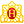Heritage Bank

«Term Deposits»

0,90

interest rate, %

rate type

fixed

interest periodicity

at maturity

minimum sum

\$ 1 000,00

6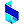Bank of Sydney

«Term Deposit»

0,90

interest rate, %

rate type

fixed

interest periodicity

at maturity

minimum sum

\$ 1 000,00

7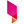Bank Australia

«Fixed term deposits»

0,90

interest rate, %

rate type

fixed

interest periodicity

at maturity

minimum sum

\$ 500,00

8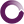Beyond Bank Australia

«monEsaver Account»

0,85

interest rate, %

rate type

variable

interest periodicity

monthly

minimum sum

\$ 0,00

9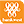Bank of Western Australia

0,80

interest rate, %

rate type

fixed

interest periodicity

at maturity

minimum sum

\$ 10 000,00

10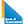Bank of Queensland

«Fixed deposit»

0,75

interest rate, %

rate type

fixed

interest periodicity

at maturity

minimum sum

\$ 5 000,00

11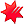National Australia Bank

«NAB Term Deposits»

0,70

interest rate, %

rate type

fixed

interest periodicity

at maturity

minimum sum

\$ 5 000,00

12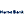Hume Bank

«Term Deposit Account»

0,70

interest rate, %

rate type

fixed

interest periodicity

at maturity, annually

minimum sum

\$ 5 000,00

13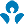Australia and New Zealand (ANZ)

0,65

interest rate, %

rate type

fixed

interest periodicity

monthly

minimum sum

\$ 0,00

14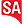Bank SA

«Fixed Term Deposit»

0,55

interest rate, %

rate type

fixed

interest periodicity

at maturity

minimum sum

\$ 1 000,00

15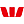Westpac Bank

0,55

interest rate, %

rate type

fixed

interest periodicity

at maturity, annually

minimum sum

\$ 5 000,00

16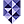Bank of Melbourne

«Fixed Term Deposit»

0,55

interest rate, %

rate type

fixed

interest periodicity

at maturity

minimum sum

\$ 1 000,00

17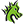St. George Bank

0,55

interest rate, %

rate type

fixed

interest periodicity

at maturity

minimum sum

\$ 1 000,00

18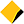Commonwealth Bank of Australia

«Term deposit»

0,50

interest rate, %

rate type

fixed

interest periodicity

at maturity

minimum sum

\$ 50 000,00

19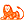ING Bank

0,50

interest rate, %

rate type

fixed

interest periodicity

at maturity

minimum sum

\$ 10 000,00

To view the up-to-date rating of the deposits at our website here.